# Optimization Problems Applied Minimum and Maximum Problems Maximum

• Slides: 11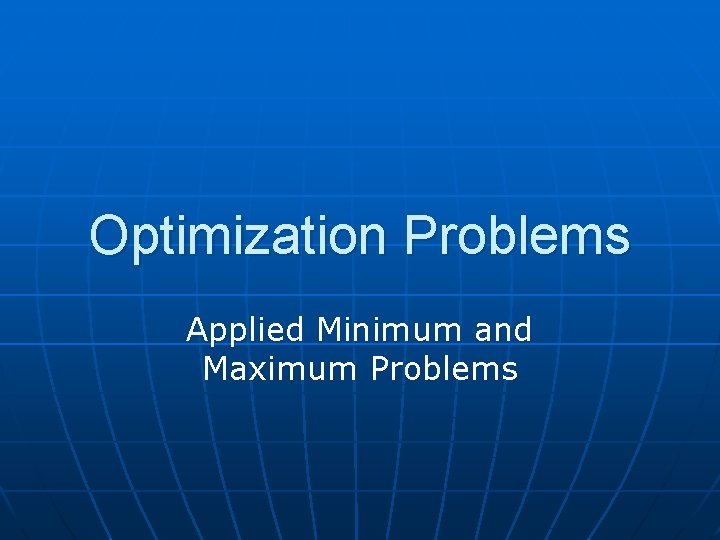Optimization Problems Applied Minimum and Maximum ProblemsMaximum Volume n A manufacturer wants to design an open box having a square base and a surface area of 108 sq. in. What dimensions will produce a box with maximum volume?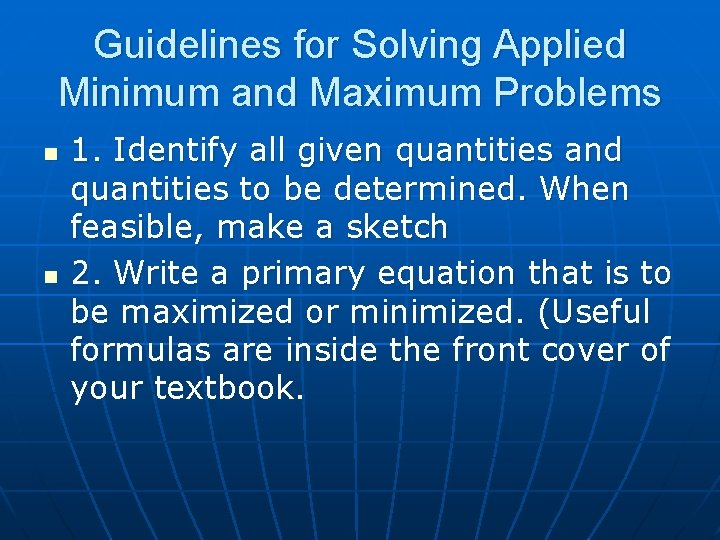Guidelines for Solving Applied Minimum and Maximum Problems n n 1. Identify all given quantities and quantities to be determined. When feasible, make a sketch 2. Write a primary equation that is to be maximized or minimized. (Useful formulas are inside the front cover of your textbook.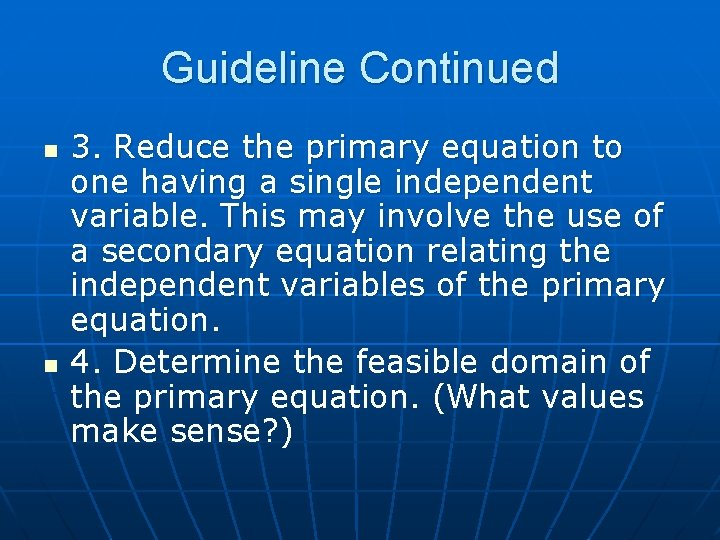Guideline Continued n n 3. Reduce the primary equation to one having a single independent variable. This may involve the use of a secondary equation relating the independent variables of the primary equation. 4. Determine the feasible domain of the primary equation. (What values make sense? )Guidelines Continued n n 5. Determine the desired maximum or minimum value by doing the derivative and setting it equal to zero. 6. Make sure the value is the desired value (max or min) by using the second derivative test.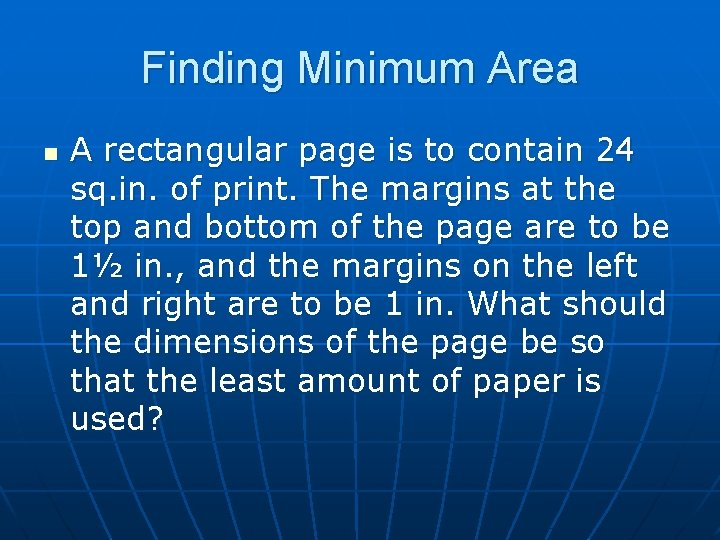Finding Minimum Area n A rectangular page is to contain 24 sq. in. of print. The margins at the top and bottom of the page are to be 1½ in. , and the margins on the left and right are to be 1 in. What should the dimensions of the page be so that the least amount of paper is used?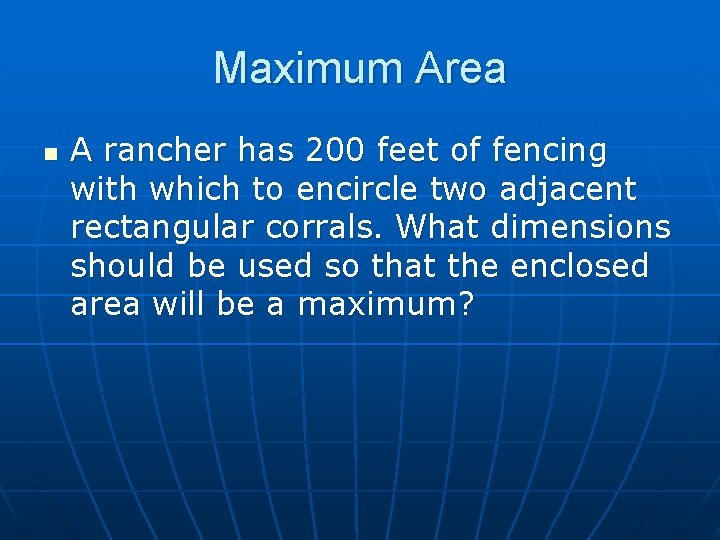Maximum Area n A rancher has 200 feet of fencing with which to encircle two adjacent rectangular corrals. What dimensions should be used so that the enclosed area will be a maximum?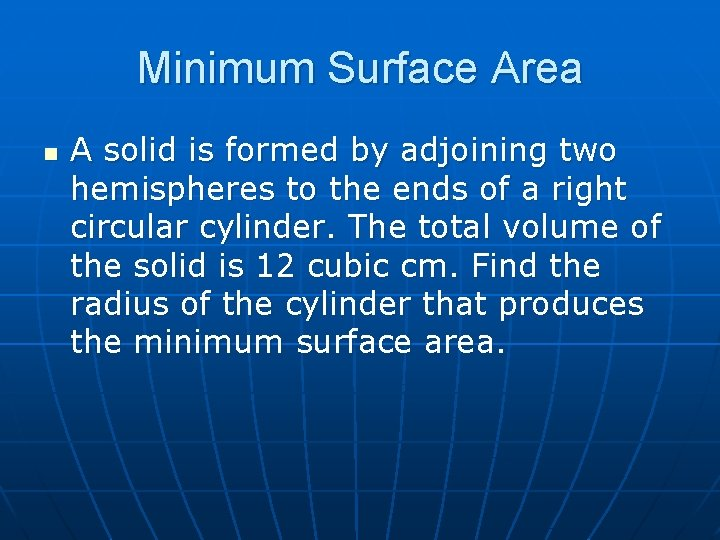Minimum Surface Area n A solid is formed by adjoining two hemispheres to the ends of a right circular cylinder. The total volume of the solid is 12 cubic cm. Find the radius of the cylinder that produces the minimum surface area.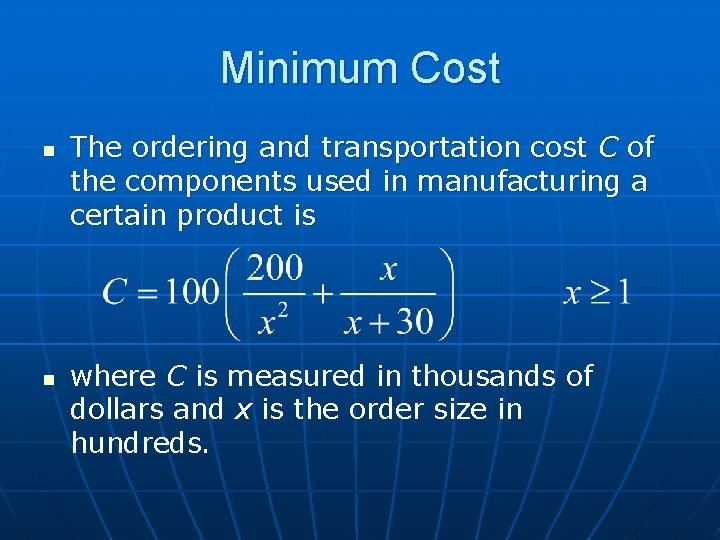Minimum Cost n n The ordering and transportation cost C of the components used in manufacturing a certain product is where C is measured in thousands of dollars and x is the order size in hundreds.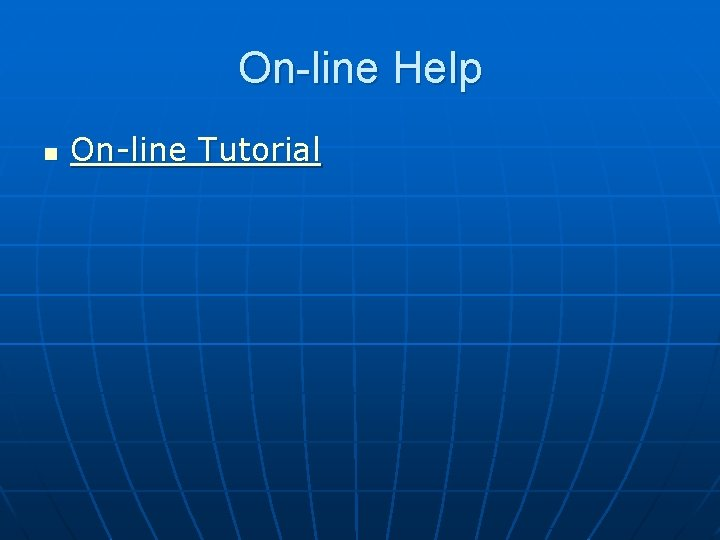On-line Help n On-line TutorialYour Turn n Develop a Optimization Problem using the topic that you provided to me when we began this lesson.